# Noise Figure Measurements VectorStar

### Making successful, confident NF measurements on Amplifiers

The Anritsu VectorStar's Noise Figure – Option 041 enables the capability to measure noise figure (NF), which is the degradation of the signal-to-noise ratio caused by components in a signal chain. The NF measurement is based on a cold source technique for improved accuracy. Various levels of match and fixture correction are available for additional enhancement. VectorStar is the only VNA platform offering a Noise Figure option enabling NF measurements from 70 kHz to 125 GHz. It is also the only VNA platform available with an optimized noise receiver for measurements from 30 to 125 GHz.

 NF Overview NF Definition Importance of Accurate NF Measurements NF Measurement Methods Y-Factor / Hot-Cold Noise Figure Measurement Method Cold-Source Noise Figure Measurement Method NF Measurement Process Test Preparation / Setup Instrument Setup Measurement Procedure Measurement Example Uncertainties Uncertainty Calculator Calculation Example Measurement Tips and Considerations References / Additional Resources
1. NF Overview
1. NF Definition
Noise figure is a figure-of-merit that describes the degradation of signal-to-noise ratio (SNR) due to added output noise power (No) of a device or system when presented with thermal noise at the input at standard noise temperature (T0, typically 290 K). For amplifiers, the concept can be readily seen. Ideally, when a noiseless input signal is applied to an amplifier, the output signal is simply the input multiplied by the amplifier gain. Realistically, the input signal is not noiseless and the output of the amplifier is larger than the input signal multiplied by the gain because noise is added through the process of amplification. Noise figure describes this relationship.

Noise factor (F) of a system is defined as:
F = (Si/Ni) / (So/No), where
Si = Input signal power
Ni = Input noise power
So = Output signal power
No = Output noise power
Noise figure (NF) is:
NF (dB) = 10 * log (F
2. Importance of Accurate NF Measurements
The addition of noise can manifest itself in many ways. In a cellular system, it can affect voice quality. In digital communications, it can affect bit-error rate, either slowing the communications or degrading quality (of a streamed video, perhaps). In radar systems, it can limit range and/or detection of small radar cross-section targets. To overcome SNR degradations, one can either increase the signal power or decrease noise. There are often constraints on increasing signal level, for example, regulatory controls, system power consumption limitations, antenna size restrictions, and system cost restraints. It is often easier and less expensive to decrease noise, rather than increase signal power. Measuring NF enables a way of comparing device or system component noise performance.

NF measurement accuracy is important for a number of reasons. With the increasing demand for greater performance being placed on so many systems (whether they are cellular, radar, digital communications,…), precisely knowing the noise performance is critical. For R&D, higher accuracy leads to better system/component models, which enables better correlation between design simulations and measurements. This can lead to better system optimization for greater product differentiation or more predictable developments for faster time-to-market. In manufacturing, better accuracy can lead to reduced measurement guard bands, which can result in tighter specifications for greater product differentiation as well as higher yields, improved throughput, and lower overall test costs.
2. NF Measurement Methods
In recent years, numerous improvements have been made in noise figure measurements through better algorithmic understanding of the measurements (e.g., -), more sensitive receivers, and less error prone methods of processing noise power measurements. Anritsu has incorporated a number of these improvements in the Noise Figure Option (Option 41) of the VectorStar platform. One of these improvements is the incorporation of the cold-source noise figure measurement method. It is one of the two common noise figure measurement methods. The other is the hot-cold or Y-factor method.
1. Y-Factor / Hot-Cold Noise Figure Measurement Method
In the past, a popular technique for noise figure measurements was termed a Y-factor or hot-cold method in which a noise source was employed that could produce a low noise output power (cold = Nc) and an elevated one (hot = Nh). This noise source becomes the 'Input Noise Signal' in Figure 1. The ratio of measured noise powers in these two states was termed the Y-factor (e.g. ) (Y = Nh/Nc) and could lead to a quick calculation of noise figure via the equation (Eq. 1) below.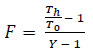Equation 1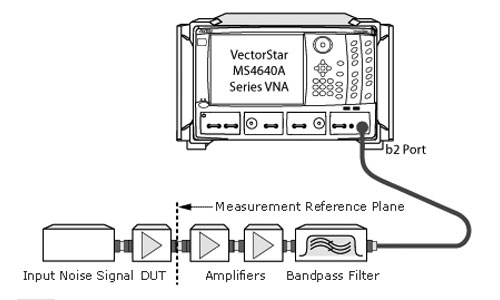Figure 1. Noise Figure Measurement Representative Diagram.

Th is the equivalent hot temperature of the noise source and the cold temperature is assumed equal to a room temperature of 290 K = T0 (per IEEE definition). By making a noise figure measurement of the receiver itself (Frcvr) and of the system (Fsys = FDUT + rcvr), it is possible to deconvolve the DUT noise figure with the familiar Friis' equation (Eq. 2) below: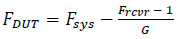Equation 2

Here the DUT gain, G, could be measured separately (via S-parameters) or it could be determined from the change in measured noise powers during calibration and measurement. One advantage the hot-cold noise figure measurement method had was that no absolute power calibrations were needed (all based on ratios). This was particularly important in the past, when wide dynamic range power measurements over large bandwidths were more difficult.
However, because of the signal levels and bandwidths involved, accurate noise source calibration (for Th) is challenging and normally left to only a few metrology laboratories. In addition to issues in calibrating the noise source, a larger challenge occurred when the match of the noise source changed between the hot and cold states . This could lead to large errors, particularly as the DUT input match worsened. If one wanted to perform a number of additional measurements, these errors could be partially corrected using a source correction method (e.g., ).

2. Cold-Source Noise Figure Measurement Method
The cold-source noise figure measurement method was developed to eliminate the requirement for a multi-state noise source, which would allow the use of a simpler, better controlled noise source (nominally a termination at room temperature). In this case, the noise figure is found from a more easily populated equation (Eq. 3), but it has some subtleties: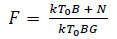Equation 3

Where:
• k = Boltzmann’s constant
• N = noise power added
• G = gain
• B = bandwidth

To calculate the noise figure, there a few key steps to accomplish. First, an absolute noise power is now required (numerator N), so a means of a receiver power calibration is needed. Anritsu’s highly accurate power calibrations and very linear broadband receiver facilitate this. Other methods are possible to determine added noise power, including the use of a calibrated hot noise source (only during the calibration step). In both cases, an absolute power reference is being created. Second, an effective measurement bandwidth (B) is needed. Since, measurement bandwidth is largely determined by the digital IF system of the VNA, B can be pre-determined. This bandwidth value may also be determined in the absolute power calibration step, if a noise source is used. Third, to isolate the noise figure of the DUT, the noise contributions of the receiver must be taken into account. As with the hot-cold method, a measurement of receiver noise is required. This time only a cold source is attached to the receiver input. Taking the receiver noise into account, Eq. 3 above can be re-expressed in the form below (Eq. 4):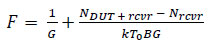Equation 4

A few things are immediately obvious. Errors in gain or the noise power measurement will propagate to noise figure on roughly a dB-for-dB basis (if the composite receiver gain is sufficiently high). These relationships will be discussed throughout this application note, but it is worth remembering the approximate dependence.

Another potential source of errors in the noise figure measurement is the interaction of the receiver with the DUT output. If the receiver noise power is strongly affected by the source impedance, then significant errors could be encountered by not accounting for this interaction. This is accomplished by error correcting with the receiver noise parameters. To understand the relationship (e.g., ), recall that the noise response of a device (receiver in this case) may be characterized by four parameters represented by Fmin, Rn, and Γopt (the latter is complex and counts as 2 parameters). Fmin is the minimum noise factor of the device when presented with an optimum source match (given by Γopt) and Rn is a sensitivity parameter describing the reaction of noise factor to changes in source match. The net noise factor of the device is then given by (Eq. 5):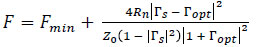Equation 4

If Rn is small relative to the system impedance Z0, then the net noise factor is relatively insensitive to changes in the source reflection coefficient, Γs. Commonly, the effective receiver Rn is small and the dependency is reduced since the receiver is normally a matched/feedback amplifier with some loss in front of it from cables, switching, etc. The noise circles of an example, typical receiver structure are shown below at 50 GHz in Figure 2. Even if the DUT output is very poorly matched (|S22| >– 1 dB), the effective receiver noise figure only changes by ≈ 0.65 dB from the minimum. Assuming the DUT has ≈ 10 dB of gain and ≈ 2 dB noise figure, this would only add 0.1 dB of uncertainty. If the DUT had ≈ 20 dB of gain and ≈ 2 dB noise figure, this would only add 0.01 dB of uncertainty. With many common receivers, even less sensitivity is displayed although the effect is, of course, a function of which pre-amplifiers are used in the composite receiver.

If further reduction in uncertainty is desirable, it is relatively straightforward to incorporate additional correction by including three additional measurements during the receiver noise calibration step (e.g., ). They are measuring the noise power with three reflect standards in addition to the cold noise source. Here one is just drawing on the long history of noise parameter measurement to help with an increase in receiver correction.

One way of measuring noise power is a dedicated noise receiver with a large, variable gain system and appropriate filtering. This can be expensive and cumbersome. Typically, these configurations have wide bandwidths, which can lead to problems with narrowband devices; errors can result if the noise measurement bandwidth is close to the DUT bandwidth and images.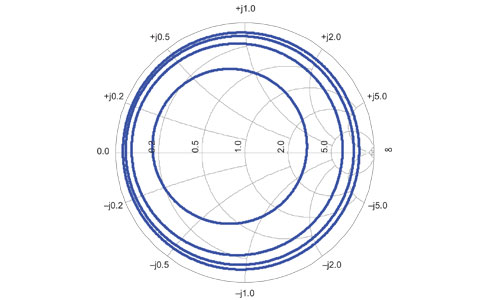Figure 2. Variable Impedance Effects on Example Receiver Noise Figure.

• NF Measurement Procedure

The noise figure measurement procedure consists of a few relatively straightforward steps:

Step 1. Measurement of DUT gain or S-parameters (over an appropriate frequency range and at an appropriate power level)
Step 2. Perform an optional user power calibration over the frequencies of interest to optimize receiver calibration accuracy
Step 3. Assembly of composite receiver for the noise figure measurement
Step 4. Receiver calibration (transferring the traceable power accuracy to the receiver)
Step 5. Basic setup (frequency range, number of points, …)
Step 6. Recall DUT gain and receiver calibration data
Step 7. Noise calibration (measurement of the receiver noise power so it can be removed from the calculations)
Step 8. DUT measurement
1. Test Preparation / Setup (Steps 1-4)
The first pre-requisite for performing a noise figure measurement is obtaining DUT gain or S-parameter data. The process of measuring DUT gain or S-parameters and saving to a *.s2p file is a standard VectorStar operation and will not be covered in this application note. For information on these topics, please see the VectorStar MS4640A Series VNA Measurement & Calibration Guide. It is important to ensure the data obtained includes the frequencies of interest for the noise figure measurement and that the device is not in a compressed state when the measurements are made.Figure 3. Basic configuration for b2 composite receiver calibration.

• The second pre-requisite is to perform a b2 receiver calibration; see Figure 3 for basic configuration. The receiver calibration must be made on the composite receiver. The required composite receiver gain is somewhat dependent on the DUT, but 40 dB of gain is a reasonable starting point. The noise figure of the composite receiver itself is generally not as critical, but less than 5 dB or so is generally desirable. If using cascaded amplifiers, the amplifier closest to the DUT dominates the composite receiver noise figure. Include a filter to isolate the LO harmonic of interest. The VectorStar MS4640C series uses harmonic downconversion. The fundamental frequency range is 2.5 to 5 GHz and 5 to 10 GHz.

It is recommended, but not required, to perform a power calibration on Port 1, prior performing the receiver calibration. The power calibration requires a power meter and sensor. Both the power and receiver calibrations are standard VectorStar operations and will not be covered in this application note. For information on these topics, please see the VectorStar MS4640A Series VNA Measurement & Calibration Guide. It is important to ensure the power calibration and receiver calibration are performed at the power level expected at the output of the DUT and the resulting power level through the user supplied amplifers in the composite receiver does not put the VectorStar receiver into compression; the VectorStar b2 receiver 0.1 dB compression point (P0.1dB) is ~–15 to –5 dBm, depending on frequency. In addition, the calibrations need to include the frequencies of interest for the noise figure measurement.

• Instrument Setup (Step 5)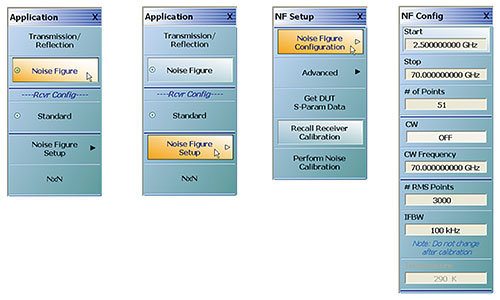Select the desired frequency range.
# of Points is the number of frequency steps within the sweep range.
# RMS Points is the number of measurements per sweep point used in the noise power computation. Trade-offs can be made on measurement speed versus data jitter by adjusting the measurement number of RMS points.
IFBW should be the same as that used in the receiver calibration.

Temperature is nominally the temperature of the cold termination used during the measurements and defaults to 290 K, specified by the IEEE definition.

• Measurement Procedure (Steps 6 - 8)
✓ Get DUT S-Param Data – This will be a *.s2p file. See Figure 4.
✓ Recall Recevier Calibration – This will be a *.rcvr file. A reminder will be shown to ensure the calibration was performed on the composite receiver. See Figure 5.
✓ Perform Noise Calibration – A diagram will be shown as a reminder of the calibration setup. See Figure 6.
After completing each task, the menu button will display a checkmark ☑.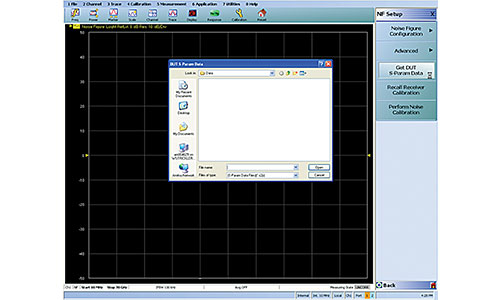Figure 4. Retrieving *.s2p DUT S-parameter file.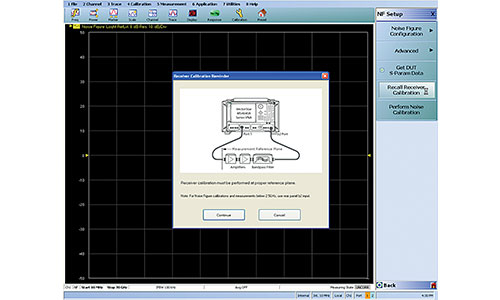Figure 5. Recalling the composite receiver calibration, a *.rcvr file.Figure 6. Performing a noise calibration.

Depending on how many frequency points are used, noise calibration may be the most time-consuming of the setup/calibration steps (allow on the order of 0.2 seconds to 2 seconds per frequency point depending on IFBW, averaging, and number of RMS points). If the frequency range is changed after calibration, interpolation and extrapolation of both the noise calibration data and DUT S-parameter data will be employed. Uncertainties may degrade, particularly if extrapolation is invoked by going outside the original frequency range of either step.

Insert the DUT and begin measuring noise figure.

NF response traces include: Noise Figure, Noise Temperature, and Noise Power.Figure 7. Basic configuration for noise figure measurement.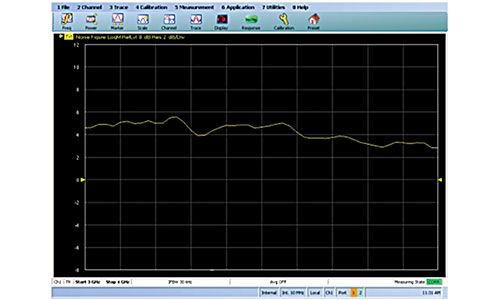Figure 8. Example noise figure trace.

• Measurement Example
To provide a measurement example, the NF of a Mini-Circuits ZJL-6G+ low power amplifier was measured between 3.5 - 4 GHz.

•  Frequency (GHz) Gain (dB) Max Power (dBm) NF (dB) VSWR (X:1) DC Power ZJL-6G+ .02 - 6 13, typ. + 10 4.5, typ. 1.5 In,1.4 Out 12 V, 50 mA

The first task was to prepare for the NF measurement.
• Measured the S-parameters of the amplifier and saved to *.s2p file.
• Determined the composite receiver requirements. Typical target is 40 dB with <≈ 5 dB noise figure. Three Mini-Circuits ZX60-5013E+ amplifiers with a gain of ≈ 14 dB and a typical NF of 3.3 dB were used, resulting in a net gain of ≈ 42 dB. NF is typically dominated by the amplifier closest to the DUT, so the composite NF was <≈ 5 dB target. In addition, an RLC F-30-4000 4 GHz low pass filter was used to minimize the noise energy outside of measurement band.
• Performed Power Calibration on Port 1. Power level was determined by selecting a power level below the 0.1 dB compression point of the VectorStar b2 receiver (≈– 5 dBm) and accounting for the composite receiver gain. The power level selected was – 10 dBm with 40 dB of source attenuation.
• Performed Receiver Calibration on the composite receiver (remembering that the calibration is performed at the b2 port) and saved to *.rcvr file.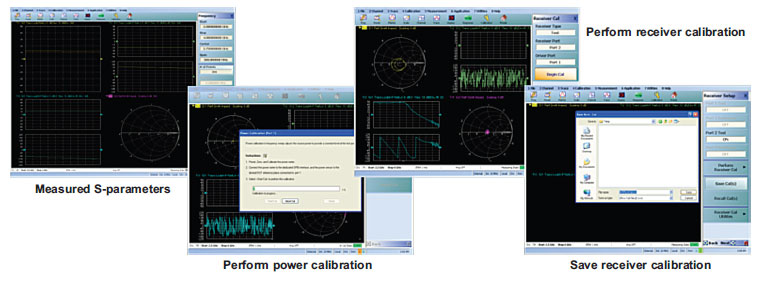Then, the NF measurement application was invoked and configured.
• Changed from Transmission/Reflection to Noise Figure mode.
• Configured the noise figure measurement with the appropriate values, including keeping the IFBW and # of points consistent with that used during S-parameter measurements and the receiver calibration.• Under the Noise Figure Setup,
– DUT S-parameters were recalled. A second trace was added showing DUT S21.
– The receiver calibration was recalled.
– A noise calibration was performed using a standard 50 Ω termination as the noise source.
– After each step, a check box ☑ appeared confirming that the task was complete.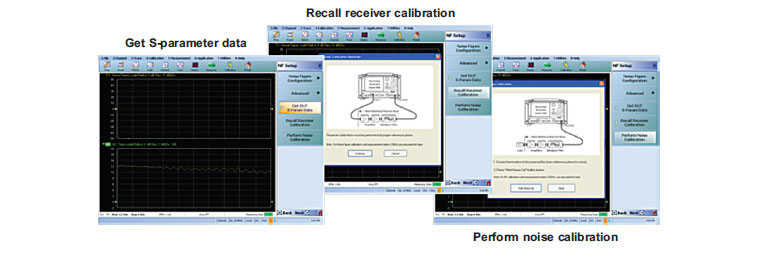Finally, the DUT was inserted and a NF measurement was made.

The expected noise figure of the Mini-Circuits ZJL-6G+ was ≈ 4.5 dB which aligned with the measurement results. The trace was saved for future reference into a *.tdf file.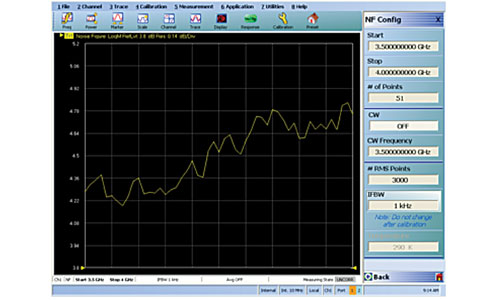1. Uncertainties

There are a number of factors affecting uncertainties. In Eq. 6 below, the Δ terms represent deviations that may occur during measurement or calibrations. Not all possible deviations are included, rather only those that are most dominant practically are shown.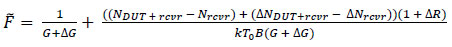Equation6

The gain variance (ΔG) is the uncertainty typically associated with s-parameter measurement. Errors due to linearity faults or other structural measurement problems are generally assumed to be taken care of by the user.

The other Δ terms relate to the measurement of noise power and are split into two groups: those common to both DUT measurement and calibration noise measurement (represented by ΔR) and those unique to the individual noise measurements (the two ΔN terms). All of these have several sub-terms which are not shown.
• ΔR: Terms related to the user power calibration (power sensor accuracy and mismatch) and to the receiver calibration (match of the receiver, match of the source, linearity and noise floor).
• ΔN: Match interactions (termination with the receiver, termination with the DUT, DUT with the receiver), power measurement linearity, and jitter.

These terms, in turn, have many dependencies on DUT S-parameters/gain, DUT noise figure, receiver noise floor, receiver linearity, source match, absolute signal levels, power sensor characteristics, etc.

typical situations, instrumentation uncertainty (ΔN) is that of the noise power measurement process and is largely data-jitter and linearity limited to ≈ 0.15 dB. There is a power calibration term (ΔR) that will vary with the calibration method used. Performing a flat power calibration followed by a receiver calibration will yield a ΔR of ≈ 0.1 dB, assuming the composite receiver return loss is > 15 dB. Using available gain and its accompanying S-parameter uncertainties lead to about 0.1 dB gain uncertainty (ΔG). The source impedance sensitivity of the receiver is typically very small and can be neglected.

Because so many variables are involved, an uncertainty calculator has been created to help analyze some of the scenarios. An increasingly preferred Monte Carlo approach (e.g., ) is used to address the random distributions (particularly in phase) of many of the variables.
1. Uncertainty Calculator
The VectorStar Noise Figure Uncertainty Calculator is a standalone program used to provide the user a tool for easily determining measurement uncertainty while using the VectorStar Noise Figure Measurement – Option 41; the calculator will operate on the desktop of VectorStar or an external PC and is an executable launched from the program menu or its icon. In addition, the calculator is helpful in determining the optimum measurement setup for achieving a desired measurement uncertainty target.

The program user interface provides editable fields for the most common noise figure measurement test setup variable parameters, such as composite receiver and DUT information. When the Calculate button is pressed, the input is used to determine the overall measurement uncertainty. Conversely, a desired DUT uncertainty value can be input and the calculator will provide a display of required receiver performance (gain) for a given DUT performance.

For additional input such as receiver, source, and power sensor match the Advanced Setup menu provides the ability for further modification of the measurement parameters.

The uncertainty calculator takes into account the 5 primary parameters of the system configuration:

2. DUT parameters
3. Power measurement parameters
4. Source parameters
5. Cold source temperature

With this information, the Uncertainty Calculator can provide plots of:

• NF Uncertainty vs. Frequency*
• NF Uncertainty vs. Gain*
• Receiver Gain vs. Frequency (to achieve a desired NF uncertainty)
* The upper and lower limit lines show the uncertainty range to a 95% confidence level.

Typical use scenario:
1. Select plot type; plot type selection activates required entries.
2. Setup system and input device parameters.
3. Press Calculate to plot uncertainties.
4. If uncertainty improvements are needed, modify the IFBW and # of RMS points to verify the current receiver gain and noise figure are adequate for the desired measurement uncertainty.
6. Alternatively, enter the desired uncertainty and compute required receiver parameters.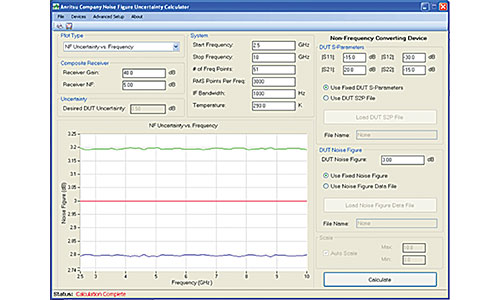• Calculation Example
The conditions from the example measurement above were used to determine the uncertainty of the NF measurement using the Anritsu NF Uncertainty Calculator. The DUT S-parameters and NF data were loaded into the calculator. With that information, it was determined that the uncertainty of the DUT NF measurement was about ± 0.2 dB over the frequency range of the measurement.
•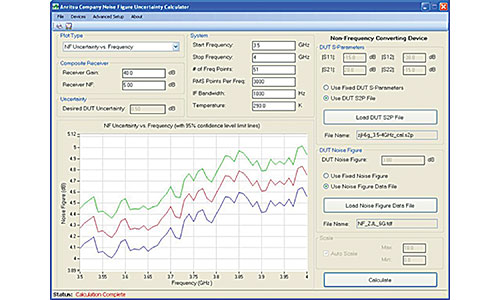Next, an investigation was conducted to determine the required gain of the composite receiver if it were desired to reduce the NF measurement uncertainty to ± 0.1 dB. The result was the gain would need increased to ≈ 60 dB. This could be accomplished by adding another Mini-Circuits ZX60-5013E+ amplifier with a gain of ≈ 14 dB to the composite receiver.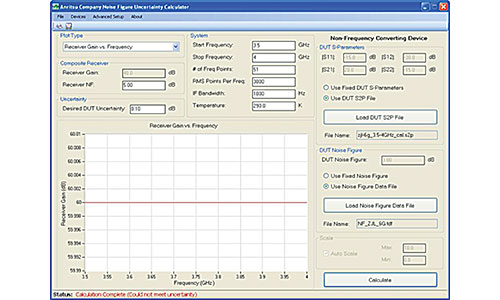• Measurement Tips and Considerations

Noise Power
• Errors in noise power measurement propagate to noise figure on roughly a dB for dB basis.
• The NF definition is based on input noise power available to the DUT and output noise power available from the DUT. As a result, available gain is more appropriate to use than insertion gain. As DUT match worsens, the difference between these gain values increases. Gain errors map essentially linearly to noise figure errors. When measuring the DUT gain/S-parameter data, a full 12-term calibration is advised for optimal accuracy and to enable the use of the more correct available gain terms. However, data from normalization-only calibrations will also be accepted.

• The downconverter is accessed through a port associated with the b2 loop. This avoids the loss associated with the coupler on Port 2 and helps improve uncertainties. Port 2 itself can be used, if the DUT + external gain is high enough (usually over 60 dB).
• The user-supplied amplifiers are used to reduce the effective composite receiver noise figure and can dramatically improve uncertainties. The required composite receiver gain is somewhat dependent on the DUT, but 40 dB of gain is a reasonable starting point. Multiple physical amplifiers can be used.
• The noise figure of the composite receiver itself is generally not as critical, but less than 5 dB or so is generally desirable. The lower the DUT noise figure, the more important the receiver amplifier noise figure becomes.
• VectorStar incorporates harmonic downconversion. By having a filter in the receiver chain to isolate the LO harmonic of interest, the other multiples can be effectively removed. Recall that any downconverter has responses for at least some harmonics of the LO (commonly 3rd, 5th, 7th… for balanced mixers and all harmonics for some structures). Thus, noise energy at an IF offset from these LO harmonics will also convert to the system IF and be included in the noise power computation. This effect becomes more pronounced when the desired product is already a harmonic of the basic LO. In that case, sub-harmonic components can play a role and they may have higher conversion efficiencies.

• If the receiver noise power is strongly affected by the source impedance and this is not taken into account, interaction between the measurement composite receiver and the DUT output can be a significant source of noise figure error. The Anritsu receiver is not strongly affected because it incorporates a well matched feedback amplifier. Sensitivity is further reduced with the use of additional amplifiers in the composite receiver. However, if further reduction in uncertainty is desirable, it is relatively straightforward to add additional correction by including three additional measurements during the receiver calibration (e.g., ).They are measuring the noise power with three reflect standards in addition to the cold noise source.
• Since a noise power measurement is over a large bandwidth and the combined gains of both the receiver and DUT may be large (> 40 dB), the integrated power may be large enough to cause compression either in the amplifiers or in the IF of the instrument. Keep in mind, the default VNA default power ranges from – 10 to + 5 dBm (depending on model, options, and frequency).
• Integral and differential ADC nonlinearities become most obvious at the lower signal levels that are implicit in a noise figure measurement. Try to keep measurement power levels as high as possible without causing compression. ADCs also contribute quantization error.
• One may rely on the factory ALC calibration to provide the power reference for the receiver calibration. It is more accurate to perform a user power calibration at the frequencies and level of interest prior to the receiver calibration to get the power accuracy roughly to the 0.1 dB level or better.

Instrument Setup

• Ensure the frequency range of the DUT gain/S-parameter data, the receiver calibration, and the noise calibration is equal to or greater than the frequency range of the desired noise figure measurement. If not, the NF measurement will utilize extrapolation, which introduces inaccuracies. Moreover, if the gain of the DUT or composite receiver changes rapidly with frequency, it is desirable to have the same number of frequency points in both the gain and NF measurement to avoid interpolation.

• Trade-offs can be made on measurement speed versus data jitter by adjusting the measurement number of RMS points (N). The default value of N is 3000. Smaller numbers can be used for greater measurement speed (at the expense of some data jitter) and larger numbers can be used for some reduction in data jitter. Values of N greater than about 7000 generally produce little benefit in data jitter reduction.

• VectorStar has a feature that it can automatically perform an IF calibration at pre-defined time intervals. The default state is for the feature to be enabled. Should an IF calibration occur during a noise figure measurement, the measurement will terminate, the calibration will be performed, and the noise figure measurement will resume from the starting point (not from the point of termination). If the user wants to avoid this scenario, it is recommended that the automatic IF calibration feature be disabled.

#### 8.0 References / Additional Resources

1. H. T. Friis, “Noise figure of radio receivers,” Proc. IRE, vol. 32, Jul. 1944, pp. 419-422.
2. “Noise Figure”, Anritsu Application Note 11410-00210, Aug. 2000.
3. D. Vondran, “Noise figure measurement: corrections related to match and gain,” Microwave Journal, Mar. 1999, pp. 22-38.
4. N. Otegi, J. M. Collantes, and M. Sayed, “Cold-source measurements for noise figure calculation in spectrum analyzers,” 67th ARFTG Conf. Dig., Jun. 2006, pp. 223-228.
5. N. Otegi, J. M. Collantes, and M. Sayed, “Receiver noise calibration for vector network analyzer,” 76th ARFTG Conf. Dig., Dec. 2010, pp. 1-5.
6. J. M. Collantes, R. D. Pollard, and M. Sayed, “Effects of DUT mismatch on the noise figure characterization: A comparative analysis of two Y-factor techniques,” IEEE Trans. Instr. And Meas., vol. 51, Dec. 2002, pp. 1150-1156.
7. L. Escotte, R. Plana, and J. Graffeuil, “Evaluation of noise parameter extraction methods,” IEEE Trans. Micr. Theory Tech., vol. 41, Mar 1993, pp. 382-387.
8. “Supplement 1 to the “Guide to the expression of uncertainty in measurement” — Propagation of distributions using a Monte Carlo method”, Joint Committee for Guides in Metrology, 2008.
Select a country/region